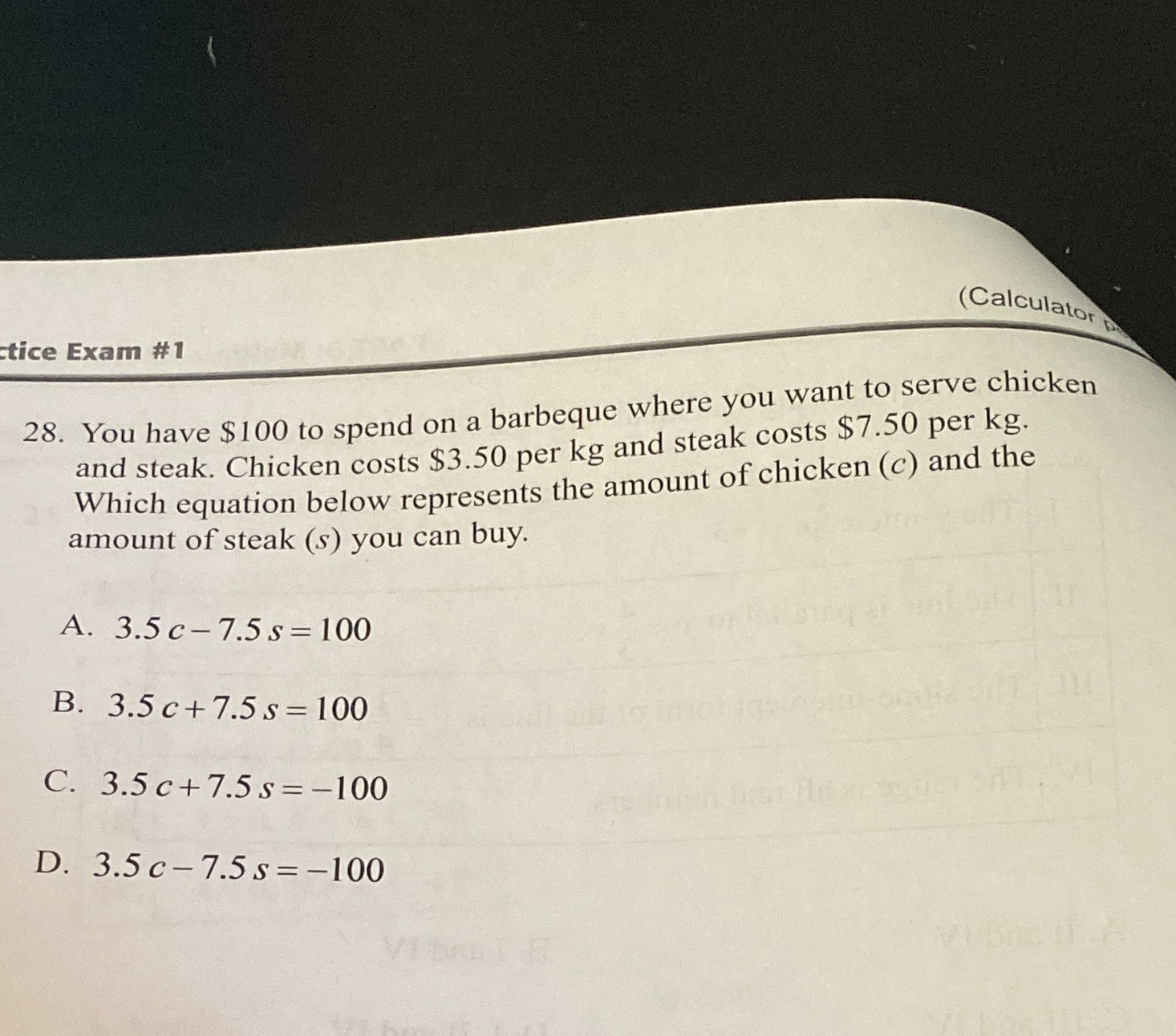### Still have math questions?

Algebra
QuestionYou have $$\ 100$$ to spend on a barbeque where you want to serve chicken and steak. Chicken costs $$\ 3.50$$ per kg and steak costs $$\ 7.50$$ per kg. Which equation below represents the amount of chicken $$( c )$$ and the amount of steak $$( s )$$ you can buy.

A. $$3.5 c - 7.5 s = 100$$

B. $$3.5 c + 7.5 s = 100$$

C. $$3.5 c + 7.5 s = - 100$$

D. $$3.5 c - 7.5 s = - 100$$